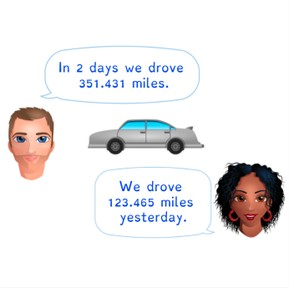Estimated and exact difference with decimal numbers with 3 decimal places

# Estimated and exact difference with decimal numbers with 3 decimal places8,000 schools use Gynzy92,000 teachers use Gynzy1,600,000 students use Gynzy

## General

Students learn to estimate and find the difference between decimal numbers with 3 decimal places.

6.NS.B.3

## Relevance

It is useful to be able to estimate and find the exact difference between decimal numbers with 3 decimal places so that you can use the estimate to check your exact answer. You can use this when you want to know about how much something weighs.

## Introduction

The interactive whiteboard has the numbers 6, 7 and a selection of decimal numbers between the two. Ask students to decide if the given decimal numbers would be rounded to 6 or to 7 and to. move them to their respective rounded numbers. Ask students to explain which number they are looking at when determining to round up or round down.

## Development

To be able to estimate the difference with decimal numbers with 3 decimal places, students must be able to round decimal numbers to whole numbers. Explain that they can do this by looking on a number line to see which whole number a decimal number is closest to. You can also explain that with larger numbers it can be easier to round to a tens or hundreds number, to make the subtraction problem easier. Next explain how to subtract numbers with 3 decimal places. Show that you can subtract on the number line when you split the subtrahend and take it away from the first number in parts. Show students that they can also subtract without a number line, by splitting both numbers and subtracting the whole numbers, subtracting the numbers after the decimal point, and then adding those differences together. Give students a few problems including a story problem discuss how you can use both methods to find the difference. Encourage students to explain their strategy.

To check that students are able to estimate the difference and find the exact difference of decimal numbers with 3 decimal places, you can ask the following questions.
- How do you round a number if you want to estimate a difference?
- How do you subtract decimal numbers if you want to know the exact difference?

## Guided practice

Students are given subtraction problems in which they are asked to estimate and then give the exact difference of decimal numbers with 3 decimal places. They are also given a story problem to estimate and find the exact difference of.

## Closing

Discuss the importance of estimating the difference of decimal numbers and how that helps to check the exact difference of decimal numbers. Ask students to solve the subtraction problems on the interactive whiteboard and to explain their strategy. Close the lesson with a few subtraction problems in which students determine how much further a car needs to ride.

## Teaching tips

Students who have difficulty with this learning goal can make use of the number line.They should first practice rounding decimal numbers to whole numbers, because then they build confidence that their estimated difference is a good check for their exact difference. You can also encourage students to take smaller jumps, or to split the subtrahend into smaller portions to take away from the minuend.

### The online teaching platform for interactive whiteboards and displays in schools

• Save time building lessons

• Manage the classroom more efficiently

• Increase student engagement